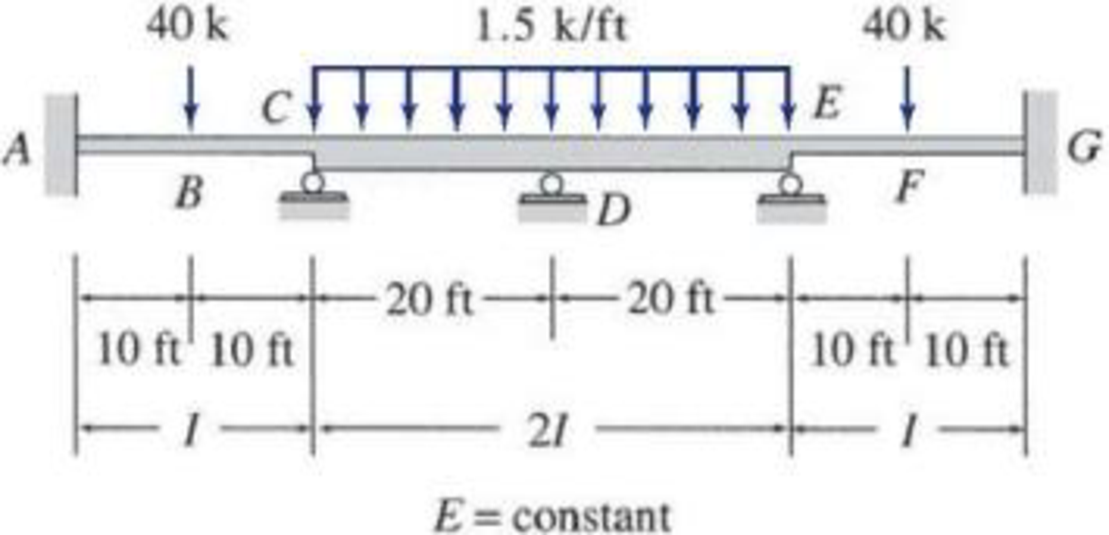# 15.8 through 15.14 Determine the reactions and draw the shear and bending moment diagrams for the beams shown in Figs. P15.8–P15.14 by using the slope-deflection method. FIG. P15.14

#### Solutions

Chapter
Section
Chapter 15, Problem 14P
Textbook Problem
10 views

## 15.8 through 15.14 Determine the reactions and draw the shear and bending moment diagrams for the beams shown in Figs. P15.8–P15.14 by using the slope-deflection method.FIG. P15.14To determine

Find the reaction and plot the shear and bending moment diagram.

### Explanation of Solution

Fixed end moment:

Formula to calculate the fixed moment for UDL is WL212.

Formula to calculate the fixed moment for the point load of equal length is PL8.

Calculation:

Consider modulus of elasticity EI of the beam is constant.

Due to symmetric loading of the beam, consider the beam DG for the analysis.

Show the free body diagram of the right half of the beam as in Figure 1.

Refer Figure 1,

Calculate the fixed end moment for DE.

FEMDE=1.5×20212=50kft

Calculate the fixed end moment for ED.

FEMED=50kft

Calculate the fixed end moment for EG.

FEMEG=40×208=100kft

Calculate the fixed end moment for GE.

FEMGE=100kft

Calculate the slope deflection equation for the member DE.

MDE=2E(2I)L(2θD+θE3ψ)+FEMDE

Substitute 0 for ψ, 0 for θD, 20 ft for L and 50kft for FEMDE.

MDE=2E(2I)20(2(0)+θE3(0))+50=0.2EIθE+50 (1)

Calculate the slope deflection equation for the member ED.

MED=2E(2I)L(θD+2θE3ψ)+FEMED

Substitute 0 for ψ, 0 for θD, 20 ft for L and 50kft for FEMED.

MED=2E(2I)20(2θE+(0)(0))50=0.4EIθE50 (2)

Calculate the slope deflection equation for the member EG.

MEG=2EIL(2θE+θG3ψ)+FEMEG

Substitute 0 for ψ, 0 for θG, 20 ft for L, and 100kft for FEMDE.

MEG=2EI20(θD+2(0)(0))+100=0.2EIθE+100 (3)

Calculate the slope deflection equation for the member GE.

MGE=2EIL(2θG+θE3ψ)+FEMGE

Substitute 0 for ψ, 0 for θG, 20 ft for L and 100kft for FEMGE.

MGE=2EI20(θE+2(0)(0))100=0.1EIθE100 (4)

Write the equilibrium equation as below.

MED+MEG=0

Substitute equation (2) and equation (3) in above equation.

0.4EIθE50+0.2EIθE+100=00.6EIθE+50=00.6EIθE=50θE=83.33EIkft2

Substitute 83.33EIkft2 for θE in equation (1).

MDE=0.2EI(83.33EI)+50=33.33kft

Substitute 83.33EIkft2 for θE in equation (2).

MED=0.4EI(83.33EI)50=83.33kft

Substitute 83.33EIkft2 for θE in equation (3).

MEG=0.2EI(83.33EI)+100=83.33kft

Substitute 83.33EIkft2 for θE in equation (4).

MGE=0.1EI(83.33EI)100=108.3kft

Show the section free body diagram of the member DE and EG as in Figure 2.

Consider the member DE of the beam:

Calculate the vertical reaction at the left end of the joint E by taking moment about point D.

+MD=0Ey,L(20)1.5×(20)×(202)+33

### Still sussing out bartleby?

Check out a sample textbook solution.

See a sample solution

#### The Solution to Your Study Problems

Bartleby provides explanations to thousands of textbook problems written by our experts, many with advanced degrees!

Get Started

Find more solutions based on key concepts
Explain traffic flow, density, and average speed.

Engineering Fundamentals: An Introduction to Engineering (MindTap Course List)

Describe types of backup used by business and home users. Explain considerations when creating a backup plan.

Enhanced Discovering Computers 2017 (Shelly Cashman Series) (MindTap Course List)

Distinguish between a PAN, LAN, MAN, and WAN.

Fundamentals of Information Systems

Convert P = 5.00 atm into Pa, bar, and psia.

Fundamentals of Chemical Engineering Thermodynamics (MindTap Course List)

What are the primary examples of public law?

Principles of Information Security (MindTap Course List)

If your motherboard supports ECC DDR3 memory, can you substitute non-ECC DDR3 memory?

A+ Guide to Hardware (Standalone Book) (MindTap Course List)

Name five different types of welding career opportunities.

Welding: Principles and Applications (MindTap Course List)